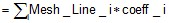# Mesh line: definition (structure)

## Definition

A mesh line is defined by:

• a name (and a comment)
• a type
• specific characteristics belonging to a type
• a color

## Name

The name to identify the mesh line is set by the user during the creation of this one.

A comment (optional) can be added to the name.

## Types of mesh lines

The different types of mesh lines and the characteristics useful for their description are gathered in the tables below.

* see next block.

Type of mesh line Description
Arithmetic Number of elements
Absolute deviation Value of the absolute deviation criterion expressed in meters determining the number of elements on a curve line
Relative deviation Value of the relative deviation criterion (0<d<1) determining the number of elements on a curve line
Geometric with imposed number of elements Number of elements Geometric progression ratio (1<R<2)
Geometric with minimum distance Length unit Minimum distance between nodes* Geometric progression ratio (1<R<2)
Length of line elements at extreme points Length unit

Length at extreme point 1*

Length at extreme point 2*

Sum of mesh lines List of associated Mesh Lines

A multiplying coefficient for each Mesh Line* see next block.

## Value

The mesh point value is defined using an algebraic expression that can contain:

• constants
• geometrical parameters
• basic mathematical functions using operators: +, -, *, /, ( )
• usual mathematical functions admitted by FORTRAN.

The mathematical functions are described in section Functions .